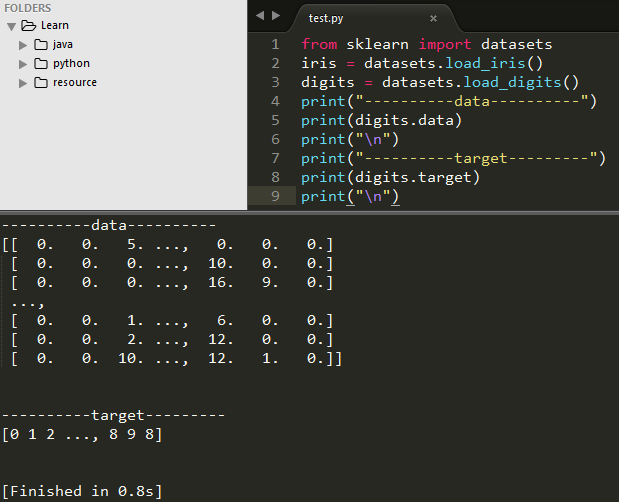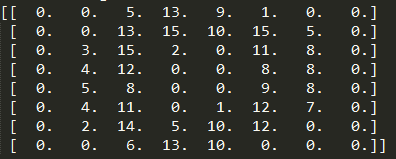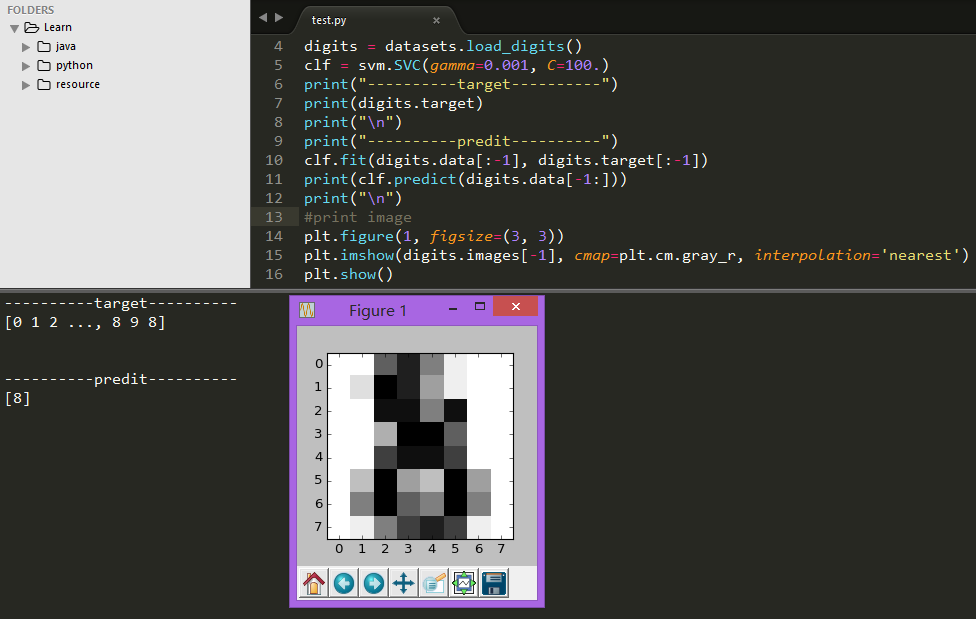# sklearn学习（2）使用scikit-learn进行机器学习的介绍

## 加载示例数据集

scikit-learn附带了一些标准数据集，例如用于分类的iris数据集和digit数据集以及用于回归的boston house prices数据集。

from sklearn import datasets

digits.data
digits.target### 数组的shape（形状）属性

digits.images### 学习及预测

from sklearn import svm
clf = svm.SVC(gamma=0.001, C=100.)

```clf.fit(digits.data[:-1], digits.target[:-1])
OUTPUT：
SVC(C=100.0, cache_size=200, class_weight=None, coef0=0.0,
decision_function_shape=None, degree=3, gamma=0.001, kernel='rbf',
max_iter=-1, probability=False, random_state=None, shrinking=True,
tol=0.001, verbose=False)```### 默认规则

scikit-learn评估器遵循某些规则，使他们的行为更具预测性。

## 发布者